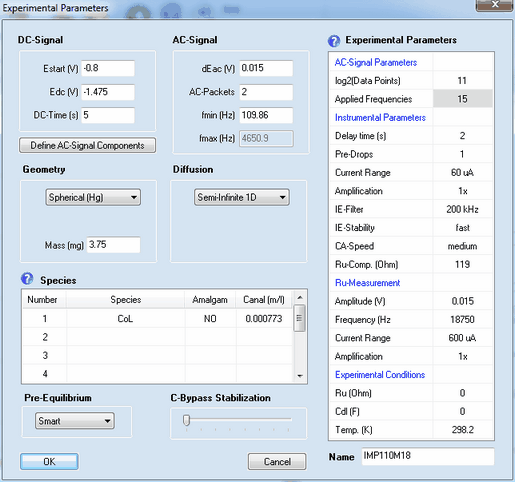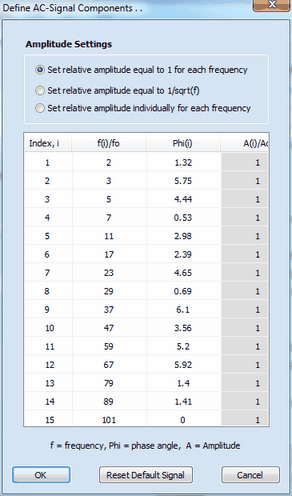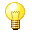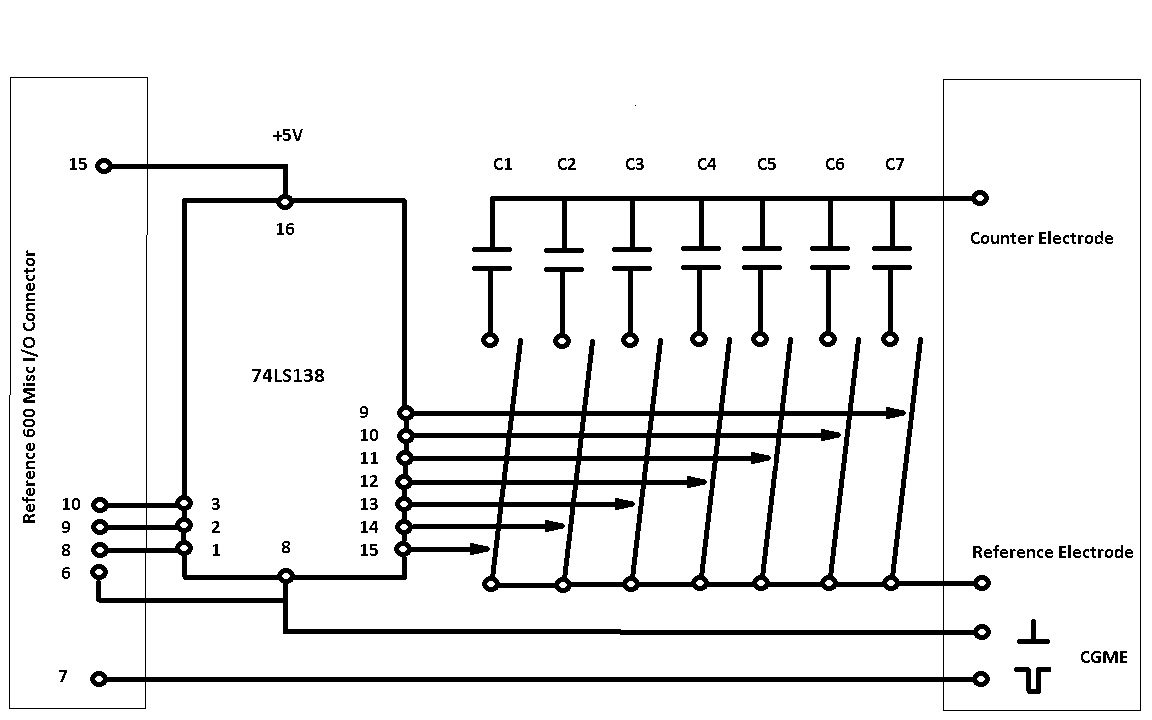﻿ Menu Command: Edit
DigiElch Help

# Menu Command: Edit# Menu Command: EditThis command opens a dialog box that enables the user to specify the following parameters referring to the active experiment:Most of the parameters have exactly the same meaning as already described for cyclic voltammetry. The meaning and functionality of the remaining parameter is as follows:

## 1. DC - Signal

Estart (V), Edc (V), DC-Time (s)
The experiment is executed in the following way: at the beginning of the experiment the potential of the electrode is assumed to be at Estart (V) so that  the initial concentrations are in agreement with the Pre-Equilibrium setting computed for this potential. Then the electrode potential jumps to Edc (V) and remains at this value for the time indicated by DC-Time (s) before the AC-Signal is superimposed.

## 2. AC-Signal Parameters

log2(Data Points)
The number of data points used for generating the AC-signal. It must be an integer power of 2 and is 2^11 = 2048 by default.

Applied Frequencies
Number of  frequency components from which the multi-sine AC-signal is generated.

## 3. AC - Signal

dEac (V), AC-Packets, fmin (Hz), fmax (Hz)
The AC-Signal can be composed of up to 15 frequencies components which can be edited/defined by clicking on the button Define AC-Signal ComponentsThe AC-signal used in the experiment can be composed of up to 15 frequency components defined by frequency, fi,  phase angle, φi, and amplitude, Ai. The values for frequency and amplitude are relative values referring to the basic frequency, fo  (i.e. the frequency which would be obtained if the signal formed by the 2n data points (where n is the number defined in log2(Data Points)) covers exactly a single sinus cycle.) The overall AC-Signal is generated using the number of frequency components defined in Applied Frequencies. Then the amplitude of the overall signal is adjusted such as to mach the value defined in dEac (V) and the time regime is adjusted in such a way that the lowest frequency component is equal to fmin (Hz). The "real world" value of the  highest frequency component, fmax (Hz), depends on the number of Applied Frequencies and the value entered for fmin (Hz). The overall AC-Signal is cyclically applied n-times where n is the number defined in AC-Packets. Consequently, AC-Packets = 2 means that the overall signal is applied twice in the course of the simulation while the Fourier-Transformation is always executed for the last of the applied AC-Packets.
It is assumed the experiment starts with jumping the potential from Estart (V) to Edc (V). The potential remains at this value for the time interval given by DC-Time(s). Then the AC-Signal is repeatedly superimposed where the number of repetitions is given by AC-Packets. The impedance data are computed from the current referring to the last AC-Packet and the applied electrode potential.

## 4. Pre-Equilibrium

Disabled , Enabled, Smart
When using the experimental CV in a Data Fitting Project the simulation(s) will be forced to be executed for the selected Pre-Equilibrium option.

## 5. Diffusion

Semi-Infinite 1D, Finite 1D, Semi-Infinite 2D
When using the experimental CV in a Data Fitting Project the simulation(s) will be forced to be executed for the selected modus of Diffusion.

## 6. Geometry

When using the experimental CV in a Data Fitting Project the simulation(s) will be forced to be executed for the selected Geometry.

## 7. Experimental Parameters

### Instrumental Parameters

Delay time (s)
The time elapsing before starting the experiment. When working with an automated mercury drop electrode a delay time of a few second is required to minimize the convection effected by the growth of the mercury drop.

Pre-Drops
When working with an automated mercury drop electrode n+1 mercury drops are produced (where n is the number of Pre-Drops) but the experiment is executed only at the last drop. Helps to improve the reproducibility of the produced mercury drops.

Current Range
The Current Range of the Reference 600 potentiostat used in the experiment. (see Reference 600 manual for more details)

Amplification
The factor with which the originally measured current signal is amplified by the Reference 600 hardware. (see Reference 600 manual for more details)

IE-Filter
Setting of the hardware filter applied to the originally measured current signal. (see Reference 600 manual for more details)

IE-Stability
The stability setting (applied to the current-to-voltage-converter) of the Reference 600 potentiostat (see Reference 600 manual for more details)

CA-Speed
The speed setting of the control-amplifier in the Reference 600 potentiostat (see Reference 600 manual for more details)

Ru-Compensation (Ohm)
Value of the ohmic resistance that will be compensated for by positive feedback (from the output of the current-to-voltage-converter to the input of the control-amplifier).

### Ru-Measurement

Amplitude (V)
Amplitude of the AC-Signal used for measuring the uncompensated ohmic resistance, Ru (Ohm), and the double layer capacity, Cdl (F).

Frequency (Hz)
Frequency of the AC-Signal used for measuring the uncompensated ohmic resistance, Ru (Ohm), and the double layer capacity, Cdl (F).

Current Range
The Current Range of the Reference 600 potentiostat with which the measurement of the uncompensated ohmic resistance, Ru (Ohm), and the double layer capacity, Cdl (F) is started. Will be automatically optimized.

Amplification
The post amplification factor used for measuring the uncompensated ohmic resistance, Ru (Ohm), and the double layer capacity, Cdl (F).

### Experimental Conditions

Ru (Ohm)
Uncompensated ohmic resistance which has been measured or entered. When using the experimental CV in a Data Fitting Project the simulation(s) will be forced to be executed for an uncompensated ohmic resistance equal to Ru (Ohm) - Ru-Compensation (Ohm).

Cdl (F)
The double layer capacity which has been measured or entered.

Temp (K)
When using the experimental CV in a Data Fitting Project the simulation(s) will be forced to be executed for this temperature.The values entered for Ru (Ohm) and Cdl (F) will be overwritten each time when executing the Command: Measure IR-Drop & Run or IR-Drop.

## 8. Species

Click on the first empty input field headlined Species to add a name for each species for which the analytical concentration is explicitly known when running the experiment(s). When using the experimental CV in a Data Fitting Project the simulation(s) will be forced to use the entered analytical concentration provided a species with exactly the same name is found in the mechanism of the Data Fitting Project.

## 9. C-Bypass Stabilization

The stabilization level adjusted at this slider bar takes effect only if the reference and counter electrode of the Reference 600 potentiostat are connected by a switchable capacitor shown as shown in the following pictureThe following capacitors can be recommended:

C1 0.1 nF

C2 0.22 nF

C3 0.44 nF

C4 1 nF

C5 2.2 nF

C6 4.7nF

C7 10 nF

The optimal value is found by "trial and error". It enables the user to conduct fully IR-compensated CV experiments using scan rates higher than 100 V/s in combination with the CA-Speed level "very fast".

Line 7 of the Reference 600 Misc I/O Connector can be used to trigger the generation of a mercury drop, for instance, by means of the Controlled Growth Mercury Electrode (BASi).